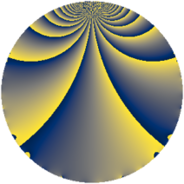# Properties

 Label 3332.1.gLevel $3332$ Weight $1$ Character orbit 3332.g Rep. character $\chi_{3332}(883,\cdot)$ Character field $\Q$ Dimension $19$ Newform subspaces $9$ Sturm bound $504$ Trace bound $13$

# Related objects

## Defining parameters

 Level: $$N$$ $$=$$ $$3332 = 2^{2} \cdot 7^{2} \cdot 17$$ Weight: $$k$$ $$=$$ $$1$$ Character orbit: $$[\chi]$$ $$=$$ 3332.g (of order $$2$$ and degree $$1$$) Character conductor: $$\operatorname{cond}(\chi)$$ $$=$$ $$68$$ Character field: $$\Q$$ Newform subspaces: $$9$$ Sturm bound: $$504$$ Trace bound: $$13$$

## Dimensions

The following table gives the dimensions of various subspaces of $$M_{1}(3332, [\chi])$$.

Total New Old
Modular forms 38 29 9
Cusp forms 22 19 3
Eisenstein series 16 10 6

The following table gives the dimensions of subspaces with specified projective image type.

$$D_n$$ $$A_4$$ $$S_4$$ $$A_5$$
Dimension 19 0 0 0

## Trace form

 $$19 q - q^{2} + 9 q^{4} - q^{8} + 17 q^{9} + O(q^{10})$$ $$19 q - q^{2} + 9 q^{4} - q^{8} + 17 q^{9} + 2 q^{13} + 9 q^{16} - q^{17} - 7 q^{18} - q^{25} - 2 q^{26} - 10 q^{30} + 9 q^{32} + q^{34} - 3 q^{36} - 11 q^{50} + 2 q^{52} - 6 q^{53} - 10 q^{60} + 9 q^{64} - q^{68} - 17 q^{72} + 11 q^{81} + 10 q^{86} + 2 q^{89} - 8 q^{93} + O(q^{100})$$

## Decomposition of $$S_{1}^{\mathrm{new}}(3332, [\chi])$$ into newform subspaces

Label Dim $A$ Field Image CM RM Traces $q$-expansion
$a_{2}$ $a_{3}$ $a_{5}$ $a_{7}$
3332.1.g.a $1$ $1.663$ $$\Q$$ $D_{2}$ $$\Q(\sqrt{-1})$$, $$\Q(\sqrt{-17})$$ $$\Q(\sqrt{17})$$ $$-1$$ $$0$$ $$0$$ $$0$$ $$q-q^{2}+q^{4}-q^{8}-q^{9}+2q^{13}+q^{16}+\cdots$$
3332.1.g.b $1$ $1.663$ $$\Q$$ $D_{3}$ $$\Q(\sqrt{-17})$$ None $$1$$ $$-1$$ $$0$$ $$0$$ $$q+q^{2}-q^{3}+q^{4}-q^{6}+q^{8}-q^{11}+\cdots$$
3332.1.g.c $1$ $1.663$ $$\Q$$ $D_{3}$ $$\Q(\sqrt{-17})$$ None $$1$$ $$-1$$ $$0$$ $$0$$ $$q+q^{2}-q^{3}+q^{4}-q^{6}+q^{8}+q^{11}+\cdots$$
3332.1.g.d $1$ $1.663$ $$\Q$$ $D_{3}$ $$\Q(\sqrt{-17})$$ None $$1$$ $$1$$ $$0$$ $$0$$ $$q+q^{2}+q^{3}+q^{4}+q^{6}+q^{8}-q^{11}+\cdots$$
3332.1.g.e $1$ $1.663$ $$\Q$$ $D_{3}$ $$\Q(\sqrt{-17})$$ None $$1$$ $$1$$ $$0$$ $$0$$ $$q+q^{2}+q^{3}+q^{4}+q^{6}+q^{8}+q^{11}+\cdots$$
3332.1.g.f $2$ $1.663$ $$\Q(\sqrt{3})$$ $D_{6}$ $$\Q(\sqrt{-17})$$ None $$-2$$ $$0$$ $$0$$ $$0$$ $$q-q^{2}-\beta q^{3}+q^{4}+\beta q^{6}-q^{8}+2q^{9}+\cdots$$
3332.1.g.g $2$ $1.663$ $$\Q(\sqrt{3})$$ $D_{6}$ $$\Q(\sqrt{-17})$$ None $$-2$$ $$0$$ $$0$$ $$0$$ $$q-q^{2}+\beta q^{3}+q^{4}-\beta q^{6}-q^{8}+2q^{9}+\cdots$$
3332.1.g.h $2$ $1.663$ $$\Q(\sqrt{-1})$$ $D_{2}$ $$\Q(\sqrt{-1})$$, $$\Q(\sqrt{-119})$$ $$\Q(\sqrt{119})$$ $$2$$ $$0$$ $$0$$ $$0$$ $$q+q^{2}+q^{4}-iq^{5}+q^{8}-q^{9}-2iq^{10}+\cdots$$
3332.1.g.i $8$ $1.663$ $$\Q(\zeta_{20})$$ $D_{10}$ $$\Q(\sqrt{-119})$$ None $$-2$$ $$0$$ $$0$$ $$0$$ $$q-\zeta_{20}^{6}q^{2}+(\zeta_{20}-\zeta_{20}^{9})q^{3}-\zeta_{20}^{2}q^{4}+\cdots$$

## Decomposition of $$S_{1}^{\mathrm{old}}(3332, [\chi])$$ into lower level spaces

$$S_{1}^{\mathrm{old}}(3332, [\chi]) \cong$$ $$S_{1}^{\mathrm{new}}(68, [\chi])$$$$^{\oplus 3}$$